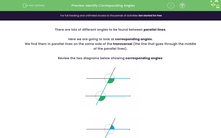# Identify Corresponding Angles

In this worksheet, students will learn how to recognise corresponding angles and use the key fact, that corresponding angles are equal, to find the value of unknown angles and solve related problems.Key stage:  KS 4

Year:  GCSE

GCSE Subjects:   Maths

GCSE Boards:   AQA, Eduqas, Pearson Edexcel, OCR,

Curriculum topic:   Geometry and Measures, Basic Geometry

Curriculum subtopic:   Properties and Constructions Angles

Popular topics:   Geometry worksheets

Difficulty level:#### Worksheet Overview

There are lots of different angles to be found between parallel lines.

Here we are going to look at corresponding angles.

We find them in parallel lines on the same side of the transversal (the line that goes through the middle of the parallel lines).

Review the two diagrams below showing corresponding angles:As we can see in the diagrams above, corresponding angles sit either below or above the parallel lines.

They also look like the letter F.

We could also say that they are in matching corners, so the word 'corresponding' can be linked to 'corners'.

The really hard part is spotting corresponding angles in diagrams.

Once we find them, we can apply the rule that these angles are always equalRight then, let's get investigating now.

In this activity, we will learn how to recognise corresponding angles and use the key fact, that corresponding angles are equal, to find the value of unknown angles and solve related problems.

### What is EdPlace?

We're your National Curriculum aligned online education content provider helping each child succeed in English, maths and science from year 1 to GCSE. With an EdPlace account you’ll be able to track and measure progress, helping each child achieve their best. We build confidence and attainment by personalising each child’s learning at a level that suits them.

Get started